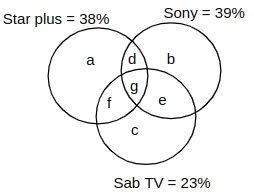Question 111

# In a survey on the viewership of the TV channels, 73% of those surveyed viewed at least one of the three Channels: Star Plus, Sab TV, and Sony. 38% of those surveyed viewed Star Plus, 39% viewed Sony, and 23% viewed Sab TV. 11% of all those surveyed viewed all the three channels. What percentage of those surveyed, viewed more than one of the three TV channels?

SolutionIt is given that 73% of those surveyed viewed at least one of the three Channels; this implies 27% viewed none of the channels
a + b + c + d + e + f + g = 73
It is given, g = 11
a + b + c + d + e + f = 62 ......... (1)
a + b + c + 2(d+e+f) + 3g = 38 + 39 + 23
a + b + c + 2(d+e+f) + 3g = 100
a + b + c + 2(d + e + f) = 67 .......... (2)
Subtracting (1) from (2), we get
d + e + f = 67 - 62 = 5
The percentage of those surveyed, viewed more than one of the three TV channels = d + e + f + g = 5 + 11 = 16

• All Quant Formulas and shortcuts PDF
• 40+ previous papers with solutions PDF